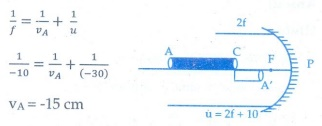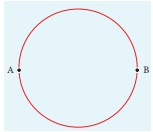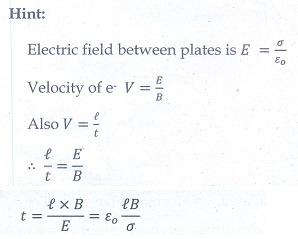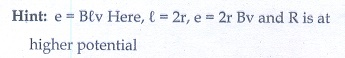# Physics - Online Test

Q1. The dimension of 1/µ0ε0 is
Explaination / Solution:Q2. A rod of length 10 cm lies along the principal axis of a concave mirror of focal length 10 cm in such a way that its end closer to the pole is 20 cm away from the mirror. The length of the image is,
Explaination / Solution:

End A of the rod acts as an object for mirror and A' will be its image. By mirror formula, image distance of A is VA.VA = -15cm

Also image distance of C

Vc= -20 cm

The length of image = | VA-VC |

= | -15-(20) | = 5cm

Q3. Two point white dots are 1 mm apart on a black paper. They are viewed by eye of pupil diameter 3 mm approximately. The maximum distance at which these dots can be resolved by the eye is, [take wavelength of light, λ = 500 nm]
Explaination / Solution:

Resolution limit = 1.22 × λ / D

D - diameter of pupil = 3 mm

λ - wavelength = 500 nm

Resolution limit = distance between the dots / resolving diameter

= 1mm/d

[ 1.22 × λ ] / D =  1mm / d

D = [1mm × D ] / [ 1.22 × λ ] = [ 1 x 10-3 × 3 x 10-3 ] / [ 1.22 × 500 x10-9 ]

d = 4.918 ≈ 5m

Q4. Which charge configuration produces a uniform electric field?
Explaination / Solution:
No Explaination.

Q5. In an electron microscope, the electrons are accelerated by a voltage of 14 kV. If the voltage is changed to 224 kV, then the de Broglie wavelength associated with the electrons would
Explaination / Solution:

deBroglie wavelength λ 1/√V

λ12 = √(V2/V1) = √(224/14) = √16 = 4

λ1 = 4λ2

λ2 = λ1/4

Q6. A wire of resistance 2 ohms per meter is bent to form a circle of radius 1m. The equivalent resistance between its two diametrically opposite points, A and B as shown in the figure isExplaination / Solution:Q7. In a hydrogen atom, the electron revolving in the fourth orbit, has angular momentum equal to
Explaination / Solution:

Angular momentum L = n h

For n = 4, L = 4h = 4h /2 π =2h/ π

Q8. An electron moves straight inside a charged parallel plate capacitor of uniform charge density σ. The time taken by the electron to cross the parallel plate capacitor when the plates of the capacitor are kept under constant magnetic field of inductionisExplaination / Solution:Q9. Doping a semiconductor results in
Explaination / Solution:
No Explaination.

Q10.

A thin semi-circular conducting ring (PQR) of radius r is falling with its plane vertical in a horizontal magnetic field B, as shown in the figure.The potential difference developed across the ring when its speed v , is
Explaination / Solution: